Analysis of Model-Calculated Soil Moisture over the United States (1931-93) and Application to Long-Range Temperature Forecasts Jin Huang, Huug van den Dool, and Konstantine P. Georgakakos -- from "Journal of Climate, Vol.9, No.6, June 1996"   2. The soil moisture model and data a. Model     Soil moisture is calculated based on the water balance in the soil. The components of the water balance in the model are precipitation, evaporation, runoff (or strearnflow divergence), and groundwater loss. We model the soil moisture to the extent it participates in landsurface processes, that is, usually in the upper I - 2 m of soil. The soil moisture budget over an area A can be expressed as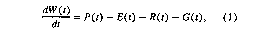where W(t) the soil water content at time t  P(t) the mean areal precipitation over area A E(t) the mean areal evapotranspiration over area A  R(t) the net strearnflow divergence from area A G(t) the net groundwater loss (through deep percolation) from area A.     The strearnflow divergence R(t) consists of a surface runoff component S(t) and a subsurface (base flow) runoff component B(t):                 R(t) = S(t) + B(t).        (2)     Following practice in operational hydrologic forecasting (e.g., Georgakakos 1986), the surface runoff and the baseflow are parameterized as follows:where Wmax is a measure of the capacity of soils to hold water in millimeters, m is a parameter with values greater than 1, a is the inverse of the response time of the baseflow, and y is a dimensionless parameter that determines the portion of the subsurface flow that becomes baseflow in the channels draining out from the area of interest. The remaining portion is lost as unobserved groundwater flow, which is then given as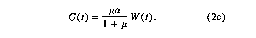The evapotranspiration E(t) is estimated in this model as follows: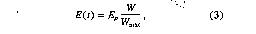where Ep is the potential evapotranspiration rate in millimeters per month. The potential evapotranspiration depends mainly on the net radiative heating on the surface. However, measurements or sufficiently accurate calculation of the net radiation on the surface (needed for aerodynamic formulas) are inadequate or absent over large areas for long times. Term Ep can also be estimated from pan evaporation, but the observations are lacking and the estimation has a number of problems (Sellers 1965). In this study, we calculate the potential evapotranspiration from the observed air temperature and duration of sunlight using Thornthwaite's method (1948). The rationale is that air temperature does, to a considerable extent, serve as a parameter of the net radiation. This is a shortcut of replacing a comprehensive atmospheric model as well as some interactions by prescribing observed temperature and precipitation.     Following Thornthwaite (1948), the formulas of E, are expressed as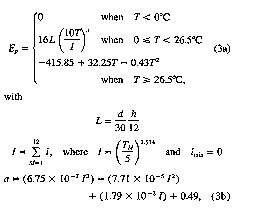where d is the number of days in the month, h the number of hours of daylight in the middle day of the month, and T the monthly mean surface air temperature in each month of the year. b. Parameter estimation from historical data     Parameter estimation (model calibration) was performed using both manual and automated search-optimization procedures. The hydrologic literature is replete with methods and applications of parameterestimation procedures to conceptual hydrologic rainfall-runoff models (e.g., reviews in Sorooshian 1991; Rajaram and Georgakakos 1989). Parameter estimation of conceptual models can be thought to be analogous to experimental calibration of assumed functional relationships in the laboratory, only with large-scale data from long historical periods. The data is used to obtain values for the parameters of the functional forms of the model that represent hydrologic processes, such as surface runoff, baseflow, etc.     Initial runs establish an initial set of parameters. The automatic downhill simplex search method implemented by Press et al. (1989) was used with modifications pertaining to the establishment of infeasible regions for the parameters. The automated search procedure estimates parameters based on the changes in the value of a quantifiable error criterion function for runoff.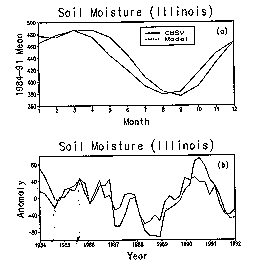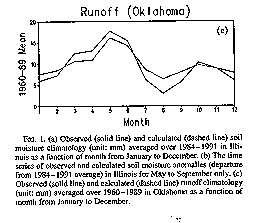The automated search procedure may converge to local optima or may lock near the boundary of the feasible region. It is then necessary to start the search from several initial parameter estimates and select the convergence region with the lowest criterion value. Once feasible parameter estimates have been obtained that possess the lowest error, runs of the model are made to examine model skill in simulating hydrologically important features of the record (i.e., magnitude and timing of flood peaks, extended low flow periods, etc.). Changes in parameter estimates may be necessary to accommodate both a low error and a good reproduction of the hydrologically important features of the runoff record. There is considerable judgement involved in the parameter estimation procedure, which requires hydrologic expertise and familiarity with model applications. C. Data and numerical procedure     The atmospheric datasets used to drive the soil model are monthly surface air temperature (T) and monthly total precipitation (P) at 344 U.S. climate divisions during the period 1931-1993. Daily station T and P data at 138 cooperative stations over 1931-1991 are also used for some specialized questions that require the use of daily data.     The observed soil moisture data in Illinois, which is the only available long-term verification dataset, are used to show the model performance. The observed soil moisture data are from 16 stations in Illinois for an 8yr period ( 1984 - 1991 ) at 11 layers of soil from the top down to 2 m in the soil (Hollinger and Isard 1994). The observed runoff data (1960-1989) from a 3' by 3' area in Oklahoma (from 34' to 37'N and 96' to 99'W) are used to calibrate the model parameters.     The ideal way to use the model for the entire United States is to estimate the model parameters for various U.S. regions of similar soil and land cover and then use these parameters for each region. In this paper, we apply the parameters estimated in Oklahoma to the entire United States and leave the model with spatially varying parameters for future work. The model parameters estimated using the Oklahoma data are Wma~ = 760 mm, p = 5.8, a = 0.093 mo-', m = 4.886, with the variables E, P, R, and G in units of millimeters per month. The model integration starts from uniform 200 mm in January 1931. The spinup time is about 3-4 months.     Because of nonlinear surface runoff generation, the integration step is varied so as not to allow the input precipitation to exceed 2 mm per time step. This technique is also used in the operational NWS Sacramento model (Peck 1974) to prevent unreasonable runoff. We use the instantaneous' soil moisture estimated by the model at the end of a month for most of the analyses and to predict future temperature. However, when comparing with observed soil moisture (in Fig. 1), we use monthly mean soil moisture.